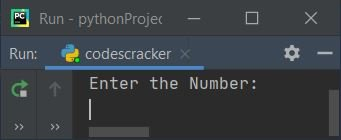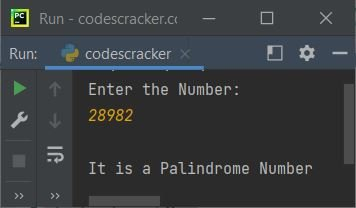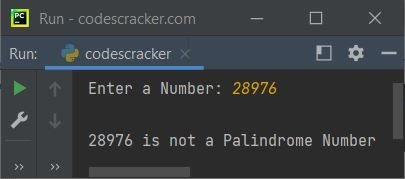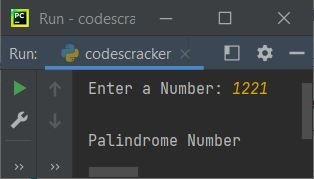# Python Program to Check Palindrome Number

In this article, we've created some programs in Python, to check whether a number entered by user is a Palindrome number or not. Here are the list of programs:

• Simple Program to Check Palindrome Number or Not
• Check Palindrome Number using Function
• Using Class

Before starting these programs, let's understand about Palindrome number in brief.

### What is a Palindrome Number ?

Palindrome number is a number whose reverse is equal to the number itself. For example, the reverse of 121 is equal to the number itself, therefore 121 can be called as a palindrome number. And 123 is not a Palindrome number, because its reverse 321 is not equal to the number itself.

## Check Palindrome Number in Python

To check whether a given number is a palindrome number or not in Python, you have to ask from user to enter a number, now reverse the number and compare it with original to check for palindrome number as shown in the program given below:

```print("Enter the Number: ")
num = int(input())
rev = 0
temp = num
while temp>0:
rem = temp%10
rev = rem + (rev*10)
temp = int(temp/10)
if rev==num:
print("\nIt is a Palindrome Number")
else:
print("\nIt is not a Palindrome Number")```

Here is the initial output produced by this Python program:Now supply the input say 28982 and press `ENTER` key to check whether it is a palindrome number or not as shown in the snapshot given below:#### Modified Version of Previous Program

In this program, the end is used to skip printing of an automatic newline. And the str() is used to convert any type of value to a string type, to concatenate string using +:

```print("Enter a Number: ", end="")
n = int(input())
rv = 0
t = n
while t>0:
rm = t % 10
rv = rm + (rv * 10)
t = int(t / 10)
if rv==n:
print("\n" + str(n) + " is a Palindrome Number")
else:
print("\n" + str(n) + " is not a Palindrome Number")```

Here is its sample run with user input, 28976:## Check Palindrome Number using Function

This program uses checkPalindrome(), a user-defined function that receives a number, and returns 1 if the reverse is equal to the number (argument's value) itself.

Therefore we've passed the value entered by user to this function as argument. So that, its return value gets initialized to chk. Therefore, while comparing the value of chk. That is, if it contains 1, means the number is a palindrome number, otherwise the number is not a palindrome number.

```def checkPalindrome(x):
t = x
v = 0
while t>0:
r = t%10
v = r + (v*10)
t = int(t/10)
if v==x:
return 1

print("Enter a Number: ", end="")
n = int(input())

chk = checkPalindrome(n)
if chk==1:
print("\n" + str(n) + " is a Palindrome Number")
else:
print("\n" + str(n) + " is not a Palindrome Number")```

This program produces similar output as of previous program.

## Check Palindrome Number using Class

This program is little similar to previous program. The only difference is, the function gets converted into a member function of the class CodesCracker. Therefore to access the member function named checkPalindrome(), we've to create an object say obj of class CodesCracker. Now use this object to access the member function using dot (.) operator:

```class CodesCracker:
def checkPalindrome(self, x):
t = x
v = 0
while t>0:
r = t%10
v = r + (v*10)
t = int(t/10)
if v==x:
return 1

print("Enter a Number: ", end="")
n = int(input())

obj = CodesCracker()
chk = obj.checkPalindrome(n)

if chk==1:
print("\nPalindrome Number")
else:
print("\nNot a Palindrome Number")```

Here is its sample run with user input, 1221:#### Same Program in Other Languages

Python Online Test

« Previous Program Next Program »